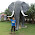# Motion | NCERT (CBSE) Class IX Science (Physics) | Multiple Choice Questions (MCQ)

Class 9, Ncert Cbse Science (Physics)
Chapter - 8, Motion
Multiple Choice Questions (MCQ)
Q.1: m/s2 is the SI unit of -
(a) distance
(b) displacement
(c) velocity
(d) acceleration.
Q.2: A car goes from a town A to another town B with a speed of 40 km/h and returns back to the town A with a speed of 60 km/h. The average speed of the car during the complete journey is -
(a) 48 km/h
(b) 50 km/h
(c) zero
(d) none of these.
Q.3: The rate of change of displacement with time is called -
(a) speed
(b) velocity
(c) acceleration
(d) retardation
Q.4: The initial velocity of a body is u. It is under uniform acceleration a. Its velocity v at any time t is given by -
(a) v = u + at2
(b) v = u + ½ at2
(c) v = u + at
(d) v = u.
Q.5: The distance covered in time t by a body having initial velocity u and having a uniform acceleration a is given by  s = ut + ½ at2. This result follows from -
(a) Newton’s first law
(b) Newton’s second law
(c) Newton’s third law
(d) None of these.
Q.6: A ball is thrown vertically upwards. It rises to a height of 50 m and comes back to the thrower,
(a) the total distance covered by the ball is zero.
(b) the net displacement of the ball is zero.
(c) the displacement is 100 m.
(d) none of these.
Q.7: In 12 minutes a car whose speed is 35 km/h travels a distance of
(a) 7 km
(b) 3.5 km
(c) 14 km
(d) 28 km
Q.8: When a graph of one quantity versus another results in a straight line, the quantities are
(a) both constant
(b) equal
(c) directly proportional
(d) inversely proportional
Q.9: A body moving along a straight line at 20 m/s undergoes an acceleration of -4 m/s2. After two seconds its speed will be -
(a) -8 m/s
(b) 12 m/s
(c) 16 m/s
(d) 28 m/s.
Q.10: A car increases its speed from 20 km/h to 30 km/h in 10 seconds. Its acceleration is
(a) 30 m/s2
(b) 3 m/s2
(c) 18 m/s2
(d) 0.83 m/s2
Q.11: A body whose speed is constant
(a) must be accelerated
(b) might be accelerated
(c) has a constant velocity
(d) can not be accelerated.
Q.12: When the distance that an object travels is directly proportional to the length of time it is said to travel with
(a) zero velocity
(b) constant speed
(c) constant acceleration
(d) uniform velocity
Q.13: a particle moves with uniform positive acceleration. Its velocity-time graph will be
(a) a straight line parallel to the time axis
(b) a straight line inclined at an obtuse angle to the time axis
(c) a straight line inclined at an acute angle to the time axis
(d) none of these.
Q.14: The slope of speed-time graph gives
(a) speed
(b) velocity
(c) acceleration
(d) momentum
Q.15: A particle experiences constant acceleration for 20 seconds after starting from rest. If it travels a distance s­1 in the first 10 seconds and distance s2 in the next 10 seconds then,
(a) s2 = s1
(b) s2 = 2s1
(c) s2 = 3s1
(d) s2 = 4s1
Q.16: In which of the following cases the object does not possess an acceleration or retardation when it moves in
(a) upward direction with decreasing speed
(b) downward direction with increasing speed
(c) with constant speed along circular path
(d) with constant speed along horizontal path
Q.17: A person travels distance πR along the circumference of a circle of radius R. Displacement of the person is
(a) R
(b) 2R
(c) 2πR
(d) zero
Q.18: The velocity of an object is directly proportional to the time elapsed. The object has
(a) uniform speed
(b) uniform velocity
(c) uniform acceleration
(d) variable acceleration

Answers: 1 - d. 2 - a. 3 - b. 4 - c. 5 - d. 6 - b. 7 - a. 8 - c. 9 - b. 10 - d. 11 - b. 12 - b 13 - c. 14 - c. 15 - c. 16 - d. 17 - b. 18 - c.

MOTION - further study

1.2.really pakao questions !

3.i want in text questions answers of chapter 8 motion

4.5.i dont like science but in physics everytime i got full mark how it is possible

6.Really!! Best questions

7.8.9.Nice for quick revison